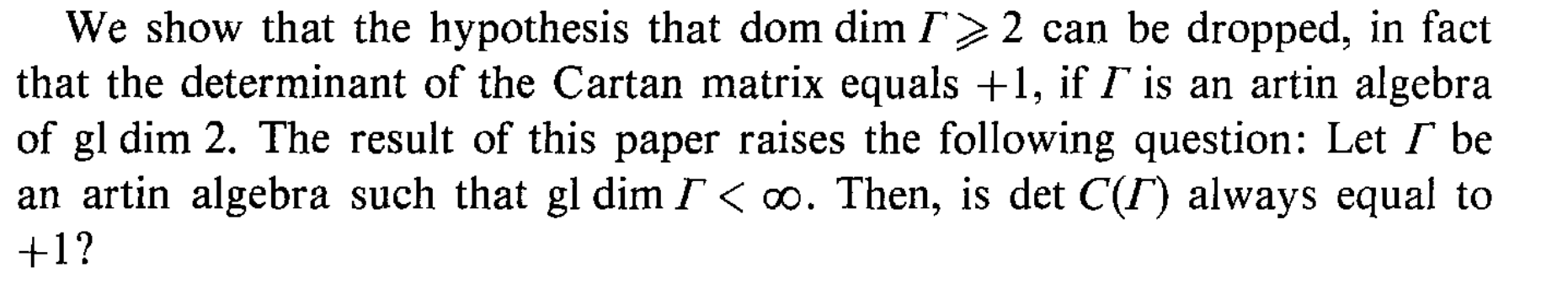# Who conjectured the Cartan determinant conjecture

The Cartan determinant conjecture states that every finite dimensional algebra of finite global dimension has the property that the determinant of its Cartan matrix is equal to one. Who stated this conjecture first and how old is it? The Cartan matrix of a finite dimensional algebra is defined as the matrix having entries $c_{i,j}:=$dimension of $(Hom_{A}(P_i,P_j))$ over the divison ring $D_i$, where $D_i:=End_A(S_i)$. Here $P_i$ are the indecomposable projective modules and $S_i$ the simple modules which are the top of $P_i$.

## 1 Answer

This seems to have first been explicitly stated as an open problem by Dan Zacharia in 1983, in On the Cartan matrix of an Artin algebra of global dimension two